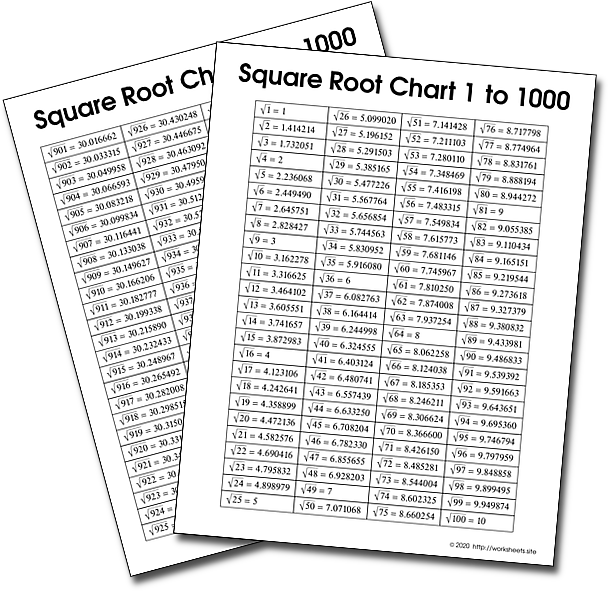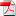# Square Root Chart for numbers 1 to 1000

Square Root Chart. Complete table of squares and square roots from numbers 1 to 1000. Square Roots Chart. Free Table of numbers Squared.Use the worksheets.site password to open the PDF file.

The perfect SQUARE ROOT of a number is the number that squared reproduces exactly the given number. Thus, 3 is the exact square root of 9 because 3 squared equals 9; 5 is the exact square root of 25 because 5 squared 2 is 25.

The German mathematician Christoff Rudolff was the one who first used the current symbol of the root, it was a corruption of the Latin word radix that means root and to denote the cubic root Rudolff repeated the sign three times this occurred in the year 1525, almost five centuries ago.

While we usually learn about square roots in the context of integers, we can also find the square roots of numbers that are not integers. For example, the square root of 10 is a decimal number close to 3.16227 (you can check this by multiplying that number times itself on a calculator and you'll get a value very close to 10.) An imperfect square is going to have a square root that is not an integer (it has a decimal or fractional part)

You can find the square roots of numbers from 1 to 1000 in the accompanying chart, with values rounded to six decimal places of precision for the roots of imperfect squares.